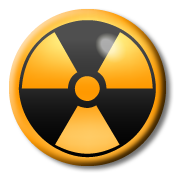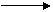1. An atom of 14 C contains protons and neutrons. 6

How many protons and neutrons are there?

 number of neutrons number of protons A. 14 6 B. 8 6 C. 6 8 D. 6 14

2+3: These questions refer to this diagram of an atom of a common element.2. What is the atomic number?

A). 3
B). 4
C). 7
D). 10

3. What is the nucleon (mass) number?

A). 3
B). 4
C). 7
D). 10

4. Two isotopes of the same element have ...

A). the same number of protons but different numbers of neutrons.
B). the same number of protons but different numbers of electrons.
C). the same number of neutrons but different numbers of protons.
D). the same number of electrons but different numbers of protons.

5. An atom that has been ionised has ...

A). Lost or gained protons
B). Lost or gained electrons
C). Lost or gained a nucleon
D). Lost or gained a neutron

6. A radioactive isotope is a substance that ...

A). will eventually gain electrons through bonding.
B). will become stable by cooling down.
C). is unstable and will ‘decay’ by losing particles.

Which type(s) of radiation match the following descriptions?7. The most ionising.
8. A fast moving electron.
9. Can pass through paper.
10. The same as a helium nucleus.

11. When Uranium decays it emits an alpha particle, forming an isotope of Thorium. Which of the following decay equations is correct?

 A. 238 U232 Th + 2 α 92 90 2
 B. 238 U236 Th + 2 α 92 90 2
 C. 238 U234 Th + 4 α 92 90 2
 D. 238 U232 Th + 4 α 92 90 2